Courses

# RD Sharma Solutions -Ex-10.1, Congruent Triangles, Class 9, Maths Class 9 Notes | EduRev

## Class 9 : RD Sharma Solutions -Ex-10.1, Congruent Triangles, Class 9, Maths Class 9 Notes | EduRev

The document RD Sharma Solutions -Ex-10.1, Congruent Triangles, Class 9, Maths Class 9 Notes | EduRev is a part of the Class 9 Course RD Sharma Solutions for Class 9 Mathematics.
All you need of Class 9 at this link: Class 9

(1) In Fig. (10).22, the sides BA and CA have been produced such that: BA = AD and CA = AE. Prove that segment DE ∥ BC.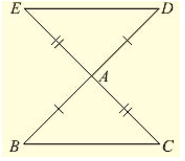Solution:

Given that, the sides BA and CA have been produced such that BA = AD and CA = AE and given to prove DE ∥ BC Consider triangle BAC and DAE,

We have

BA = AD and CA= AE                       [given in the data]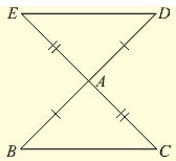And also ∠BAC = ∠DAE                                       [vertically opposite angles]

So, by SAS congruence criterion, we have

∠BAC ≃ ∠DAE

• BC = DE and ∠DEA = ∠BCA, ∠EDA = ∠CBA

[Corresponding parts of congruent triangles are equal]

Now, DE and BC are two lines intersected by a transversal DB such that ∠DEA = ∠BCA i.e.. alternate angles are equal Therefore, DE  BC ∥ BC.

Q. (2) In a PQR, if PQ = QR and L, M and N are the mid-points of the sides PQ, QR and RP respectively. Prove that LN = MN.

Solution: Given that,

In PQR, PQ = QR and L, M, N are midpoints of the sides PQ, QP and RP respectively and given to prove that LN = MN

Here we can observe that PQR is an isosceles triangle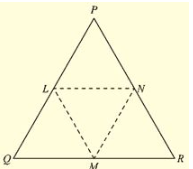• PQ = QR and ∠ QPR = ∠ QRP _____(i)

And also, L and M are midpoints of PQ and QR respectively

• PL = LQ = QM = MR =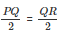And also, PQ = QR

Now, consider Δ LPN and Δ MRN, LP = MR                       [From – (2)]

∠ LPN = ∠ MRN __ [From – (1)]

∠ QPR and ∠ LPN and ∠ QRP and ∠ MRN are same.

PN = NR                                                             [N is midpoint of PR]

So, by SAS congruence criterion, we have Δ LPN = Δ MRN

• LN = MN [Corresponding parts of congruent triangles are equal]

Q. (3) In fig. (10).23, PQRS is a square and SRT is an equilateral triangle. Prove that (i) PT = QT  (ii) ∠ TQR = 15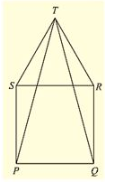Solution: Given that PQRS is a square and SRT is an equilateral triangle. And given to prove that

(i) PT =QT and (ii) ∠ TQR =15°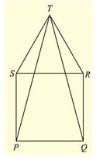Now, PQRS is a square

• PQ = QR = RS = SP ____(i)
• And ∠ SPQ = ∠ PQR = ∠ QRS = ∠ RSP = 90 = right angle

And also, SRT is an equilateral triangle.

• SR = RT = TS ___(ii)

And ∠ TSR = ∠ SRT = ∠ RTS = 60°

From (i) and (ii)

PQ = QR = SP = SR = RT = TS  ___(iii)

And also,

∠ TSP =  ∠ TSR + ∠ RSP = 60° + 90° + 150°
∠TRQ = ∠TRS + ∠ SRQ = 60° + 90° + 150°
⇒   ∠ TSR = ∠ TRQ = 150     ____ (iv)

SP = RQ           [From (iii)]

So, by SAS congruence criterion we have

Δ TSP = Δ TRQ

PT = QT   [Corresponding parts of congruent triangles are equal] Consider Δ TQR.

QR = TR        [From (iii)]

Δ TQR is a isosceles triangle.

∠ QTR = ∠ TQR [angles opposite to equal sides]

Now,

Sum of angles in a triangle is equal to 180

⇒   ∠ QTR + ∠ TQR + ∠TRQ = 180°

⇒   2 ∠ TQR + 150° = 180°     [From (iv)]

⇒   2 ∠ TQR = 180° – 150°

⇒   2 ∠ TQR = 30°                                   ∠ TQR = 15°]…

Hence proved

Q. (4) Prove that the medians of an equilateral triangle are equal.

Solution:

Given,

To prove the medians of an equilateral triangle are equal.

Median: The line Joining the vertex and midpoint of opposite side.  Now, consider an equilateral triangle ABC.

Let D,E,F are midpoints of BC, CA and AB.

Then, AD, BE and CF are medians of ABC.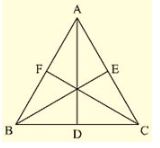Now,

D Is midpoint of BC ⇒  BD = DC =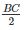Similarly, CE = EA =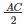AF = FB =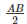Since Δ ABC is an equilateral triangle

⇒  AB = BC = CA                     _____(i)

⇒   BD = DC = CE = EA = AF = FB ===_____(ii)

And also, ∠ ABC = ∠ BCA = ∠ CAB = 60°  _____(iii)

Now, consider Δ ABD and Δ BCE AB = BC                                [From (i)]

BD = CE                               [From (ii)]

Now, in Δ TSR and Δ TRQ

TS = TR    [From (iii)]

∠ ABD = ∠ BCE            [From (iii)]           [∠ ABD and ∠ ABC and ∠ BCE and ∠BCA are same]

So, from SAS congruence criterion, we have

Δ ABD = Δ BCE

[Corresponding parts of congruent triangles are equal]

Now, consider Δ BCE and Δ CAF, BC = CA                         [From (i)]

∠ BCE = ∠ CAF             [From (ii)]

[∠ BCE and ∠ BCA and  ∠ CAF and ∠ CAB are same]

CE = AF                [From (ii)]

So, from SAS congruence criterion, we have

Δ BCE = Δ CAF

• BE = CF (v)

[Corresponding parts of congruent triangles are equal]

From (iv) and (v), we have

• Median AD = Median BE = Median CF

The medians of an equilateral triangle are equal.

Hence proved

Q. (5) In a Δ ABC, if ∠ A = 120° and AB = AC. Find ∠ B and ∠ C.

Solution:

Consider a Δ ABC

Given Mat ∠ A = 120° and AB = AC and given to find ∠ B and ∠ C.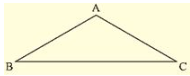We can observe that Δ ABC is an isosceles triangle since AB = AC

• ∠ B = ∠ C (i)

[Angles opposite to equal sides are equal]

We know that sum of angles in a triangle is equal to 180°

⇒  ∠ A + ∠ B + ∠ C= 180°                                                                             [From (i)]

⇒  ∠ A + ∠ B + ∠ B= 180°

⇒  120∘ + 2∠B = 180

⇒  2∠B = 180∘ – 120

⇒   ∠ B = ∠ C = 30

Q. (6) In a Δ ABC, if AB = AC and  ∠ B = 70°. Find ∠ A.

Solution:

Consider a Δ ABC, if AB = AC and  ∠ B = 70°

Since, AB = AC Δ ABC is an isosceles triangle

• ∠ B = ∠ C [Angles opposite to equal sides are equal]
• ∠ B = ∠ C = 70

And also,

Sum of angles in a triangle = 180

• ∠ A + ∠ B +∠ C = 180
• ∠ A + 70∘ + 70 = 180
• ∠ A = 180∘ – 140
• ∠ A = 40

Q. (7) The vertical angle of an isosceles triangle is (10)0. Find its base angles.

Solution:

Consider an isosceles ΔABC such that AB = AC

Given that vertical angle A is (10)0

To find the base angles

Since Δ ABC is isosceles

• ∠ B = ∠ C [Angles opposite to equal sides are equal]

And also,

Sum of interior angles of a triangle = 180

• ∠ A + ∠ B +∠ C = 180
• (10)0 + ∠ B ∠ B = 180
• 2∠ B = 180∘ – (10)0
• ∠ B = 40
• ∠ B = ∠ C = 40

Q. (8) In Fig. (10).24, AB = AC and ∠ ACD = (10)5. Find ∠ BAC.

Solution:

Consider the given figure

We have,

AB = AC and ∠ ACD = (10)5

Since, ∠ BCD = 180° = Straight angle

• ∠ BCA + ∠ ACD =180°
• ∠ BCA + (10)5° = 180°
• ∠ BCA = l80° -(10)5°
• ∠BCA= 75°

And also,

ΔABC is an isosceles triangle      [AB = AC]

• ∠ ABC = ∠ ACB [Angles opposite to equal sides are equal]

From (i), we have

∠ ACB = 75°

• ∠ ABC = ∠ ACB = 75°

And also,

Sum of Interior angles of a triangle = 180 ∘

• ∠ ABC = ∠ BCA + ∠ CAB =180°
• 75° + 75° + ∠ CAB =180°
• 150° + ∠ BAC = 180°
• ∠ BAC = 180° -150° = 30°
• ∠ BAC = 30°

Q. (9) Find the measure of each exterior angle of an equilateral triangle.

Solution:

Given to find the measure of each exterior angle of an equilateral triangle consider an equilateral triangle ABC.

We know that for an equilateral triangle

AB = BC = CA and ∠ ABC = ∠ BCA= CAB =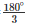= 60°                   ____(i)

Now,

Extend side BC to D, CA to E and AB to F.

Here BCD is a straight line segment

• BCD = Straight angle =180°
• ∠ BCA + ∠ ACD = 180° [From (i)]
• 60° + ∠ACD = 180°
• ∠ACD = 120°

Similarly, we can find ∠ FAB and ∠ FBC also as 120° because ABC is an equilateral triangle

∠ ACD = ∠ EAB – ∠ FBC = 120°

Hence, the median of each exterior angle of an equilateral triangle is 120°

Q. (10) If the base of an isosceles triangle is produced on both sides, prove that the exterior angles so formed are equal to each other.

Solution:

ED is a straight line segment and B and C an points on it.

• ∠ EBC = ∠ BCD = straight angle = 180°
• ∠ EBA+ ∠ ABC = ∠ ACB + ∠ ACD
• ∠ EBA = ∠ ACD + ∠ ACB – ∠ ABC
• ∠ EBA = ∠ ACD [From (i) ABC = ACD]

∠ ABE = ∠ ACD

Hence proved

Q. (11) In Fig. (10).2(5) AB = AC and DB = DC, find the ratio ∠ ABD : ∠ ACD.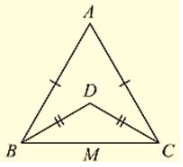Solution:

Consider the figure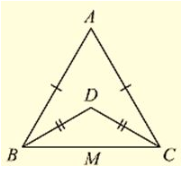Given,

AB = AC, DB = DC and given to find the ratio

∠ ABD = ∠ ACD

Now, Δ ABC and Δ DBC are isosceles triangles since AB = AC and DB = DC respectively

• ∠ ABC = ∠ ACB and ∠ DBC = ∠ DCB [Angles opposite to equal sides are equal]

Now consider,

∠ ABD : ∠ ACD

• (∠ ABC – ∠ DBC) : (∠ ACB – ∠ DCB)
• (∠ ABC – ∠ DBC) : (∠ ABC – ∠ DBC) [∠ ABC = ∠ ACB and ∠ DBC = ∠ DCB]
• 1:1

ABD: ACD = 1:1

Q. (12) Determine the measure of each of the equal angles of a right-angled isosceles triangle.

OR

ABC is a right-angled triangle in which ∠ A = 90° and AB = AC. Find ∠ B and ∠ C.

Solution:

ABC is a right angled triangle

Consider on a right – angled isosceles triangle ABC such that

∠ A = 90 and AB = AC Since,

AB = AC ⇒  ∠ C = ∠ B                                                       ____(i)

[Angles opposite to equal sides are equal]

Now, Sum of angles in a triangle = 180 ∘

∠ A + ∠ B + ∠ C =180°

⇒  90° + ∠ B+ ∠ B = 180°

⇒   2∠ B = 90°

⇒ ∠ B = 45°

∠ B = 45°, ∠ C = 45°

Hence, the measure of each of the equal angles of a right-angled Isosceles triangle Is 45°

Q. (13) AB is a line segment. P and Q are points on opposite sides of AB such that each of them is equidistant from the points A and B (See Fig. (10).26). Show that the line PQ is perpendicular bisector of AB.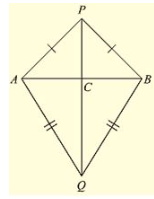Solution:

Consider the figure.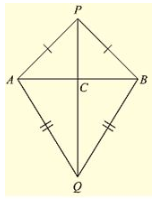We have

AB is a line segment and P, Q are points on opposite sides of AB such that

AP = BP          __________(i)

AQ = BQ        ___________(ii)

We have to prove that PQ is perpendicular bisector of AB.

Now consider Δ PAQ and Δ PBQ,

We have

AP = BP                    [From (i)]

AQ = BQ                   [From (ii)]

And PQ – PQ             [Common site]

Δ PAQ ≃ Δ PBQ     ______(iii)  [From SAS congruence]

Now, we can observe that APB and ABQ are isosceles triangles. [From (i) and (ii)]

• ∠ PAB = ∠ ABQ and ∠ QAB = ∠ QBA

Now consider Δ PAC and Δ PBC

C is the point of intersection of AB and PQ

PA = PB                       [From (i)]

∠ APC = ∠  BPC           [From (ii)]

PC = PC                        [common side]

So, from SAS congruency of triangle ΔPAC≅ΔPBC

• AC = CB and ∠ PCA = ∠ PBC _________(iv)                       [ Corresponding parts of congruent triangles are equal]
And also, ACB is line segment
• ∠ ACP + ∠ BCP = 180 ∘
• ∠ ACP = ∠ PCB
• ∠ ACP = ∠ PCB = 90∘<

We have AC = CB ⇒  C is the midpoint of AB

From (iv) and (v)

We can conclude that PC is the perpendicular bisector of AB

Since C is a point on the line PQ, we can say that PQ is the perpendicular bisector of AB.

Offer running on EduRev: Apply code STAYHOME200 to get INR 200 off on our premium plan EduRev Infinity!

91 docs

,

,

,

,

,

,

,

,

,

,

,

,

,

,

,

,

,

,

,

,

,

,

,

,

,

,

,

,

,

,

;